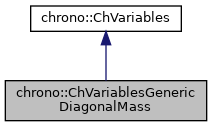chrono::ChVariablesGenericDiagonalMass Class Reference

## Description

Specialized class for representing a N-DOF item for a system, that is an item with a diagonal mass matrix and associated variables.

#include <ChVariablesGenericDiagonalMass.h>

Inheritance diagram for chrono::ChVariablesGenericDiagonalMass:[legend]
Collaboration diagram for chrono::ChVariablesGenericDiagonalMass:[legend]

## Public Member Functions

ChVariablesGenericDiagonalMass (int m_ndof=1)

ChVariablesGenericDiagonalMassoperator= (const ChVariablesGenericDiagonalMass &other)
Assignment operator: copy from other object.

ChVectorDynamicGetMassDiagonal ()
Access the diagonal mass.

virtual int Get_ndof () const override
The number of scalar variables in the vector qb (dof=degrees of freedom)

virtual void Compute_invMb_v (ChVectorRef result, ChVectorConstRef vect) const override
Computes the product of the inverse mass matrix by a vector, and add to result: result = [invMb]*vect.

virtual void Compute_inc_invMb_v (ChVectorRef result, ChVectorConstRef vect) const override
Computes the product of the inverse mass matrix by a vector, and increment result: result += [invMb]*vect.

virtual void Compute_inc_Mb_v (ChVectorRef result, ChVectorConstRef vect) const override
Computes the product of the mass matrix by a vector, and set in result: result = [Mb]*vect.

virtual void MultiplyAndAdd (ChVectorRef result, ChVectorConstRef vect, const double c_a) const override
Computes the product of the corresponding block in the system matrix (ie. More...

virtual void DiagonalAdd (ChVectorRef result, const double c_a) const override
Add the diagonal of the mass matrix scaled by c_a, to 'result'. More...

virtual void Build_M (ChSparseMatrix &storage, int insrow, int inscol, const double c_a) override
Build the mass matrix (for these variables) scaled by c_a, storing it in 'storage' sparse matrix, at given column/row offset. More...Public Member Functions inherited from chrono::ChVariables
ChVariables (int m_ndof)

ChVariablesoperator= (const ChVariables &other)
Assignment operator: copy from other object.

void SetDisabled (bool mdis)
Deactivates/freezes the variable (these variables won't be modified by the system solver).

bool IsDisabled () const
Check if the variables have been deactivated (these variables won't be modified by the system solver).

bool IsActive () const
Check if these variables are currently active. More...

ChVectorRef Get_qb ()
Returns reference to qb, body-relative part of degrees of freedom q in system: More...

virtual void Compute_fb ()
Compute fb, body-relative part of known vector f in system. More...

ChVectorRef Get_fb ()
Returns reference to fb, body-relative part of known vector f in system. More...

void SetOffset (int moff)
Set offset in global q vector (set automatically by ChSystemDescriptor)

int GetOffset () const
Get offset in global q vector.

virtual void ArchiveOUT (ChArchiveOut &marchive)

virtual void ArchiveIN (ChArchiveIn &marchive)Protected Attributes inherited from chrono::ChVariables
int offset
offset in global q state vector (needed by some solvers)

## ◆ Build_M()

 void chrono::ChVariablesGenericDiagonalMass::Build_M ( ChSparseMatrix & storage, int insrow, int inscol, const double c_a )
overridevirtual

Build the mass matrix (for these variables) scaled by c_a, storing it in 'storage' sparse matrix, at given column/row offset.

Note, most iterative solvers don't need to know mass matrix explicitly.

Implements chrono::ChVariables.

 void chrono::ChVariablesGenericDiagonalMass::DiagonalAdd ( ChVectorRef result, const double c_a ) const
overridevirtual

Add the diagonal of the mass matrix scaled by c_a, to 'result'.

NOTE: the 'result' vector must already have the size of system unknowns, ie the size of the total variables & constraints in the system; the procedure will use the ChVariable offset (that must be already updated) as index.

Implements chrono::ChVariables.

 void chrono::ChVariablesGenericDiagonalMass::MultiplyAndAdd ( ChVectorRef result, ChVectorConstRef vect, const double c_a ) const
overridevirtual

Computes the product of the corresponding block in the system matrix (ie.

the mass matrix) by 'vect', scale by c_a, and add to 'result'. NOTE: the 'vect' and 'result' vectors must already have the size of the total variables&constraints in the system; the procedure will use the ChVariable offsets (that must be already updated) to know the indexes in result and vect.

Implements chrono::ChVariables.

The documentation for this class was generated from the following files:
• /builds/uwsbel/chrono/src/chrono/solver/ChVariablesGenericDiagonalMass.h
• /builds/uwsbel/chrono/src/chrono/solver/ChVariablesGenericDiagonalMass.cpp